Electrostatic Generator Electrostatic generators convert mechanical energy directly

• Slides: 28Electrostatic Generator � Electrostatic generators convert mechanical energy directly into electrical energy. � In contrast to electromagnetic energy conversion, however, electrical charges are moved in this generator against the force of electrical fields, thus gaining higher potential energies and consuming mechanical energy. � Van de Graaff (1931) succeeded with the development of electrostatic belt-driven generators. � These generators are in common use today in nuclear physics research laboratories 1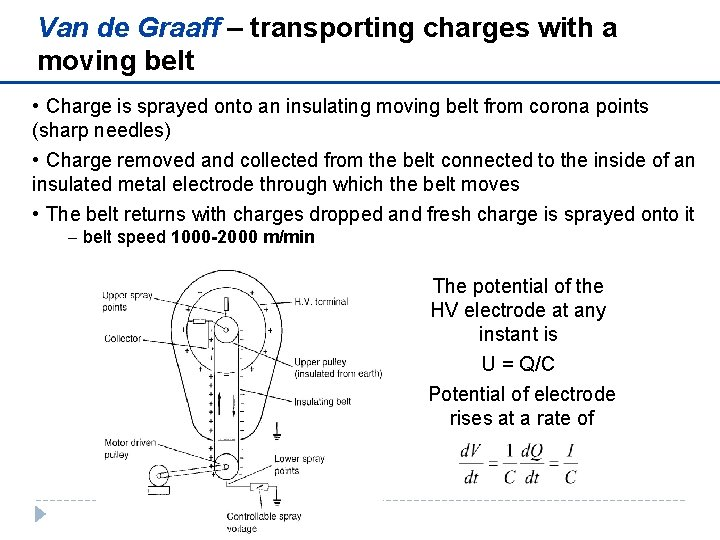Van de Graaff – transporting charges with a moving belt • Charge is sprayed onto an insulating moving belt from corona points (sharp needles) • Charge removed and collected from the belt connected to the inside of an insulated metal electrode through which the belt moves • The belt returns with charges dropped and fresh charge is sprayed onto it - belt speed 1000 -2000 m/min The potential of the HV electrode at any instant is U = Q/C Potential of electrode rises at a rate of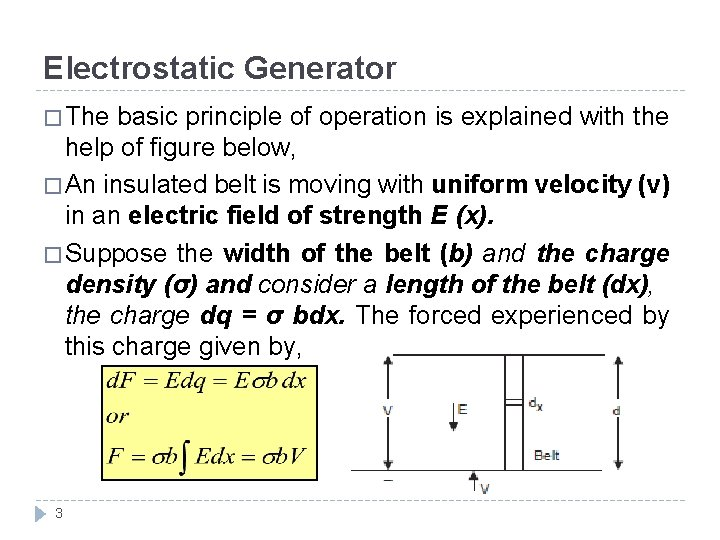Electrostatic Generator � The basic principle of operation is explained with the help of figure below, � An insulated belt is moving with uniform velocity (ν) in an electric field of strength E (x). � Suppose the width of the belt (b) and the charge density (σ) and consider a length of the belt (dx), the charge dq = σ bdx. The forced experienced by this charge given by, 3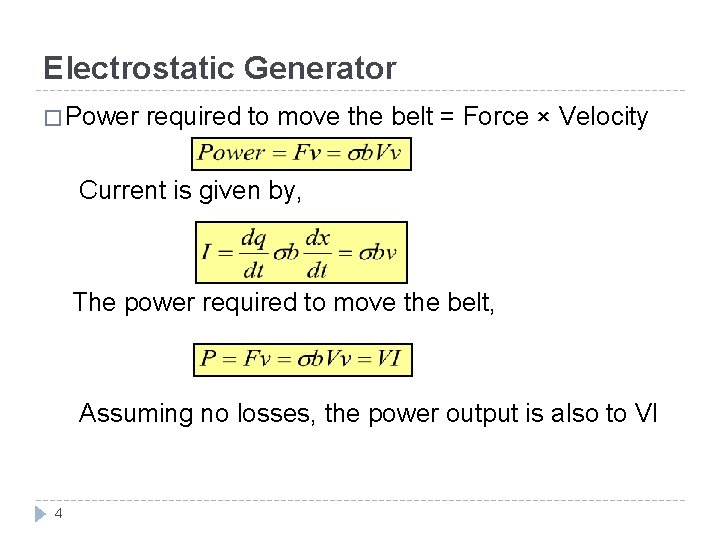Electrostatic Generator � Power required to move the belt = Force × Velocity Current is given by, The power required to move the belt, Assuming no losses, the power output is also to VI 4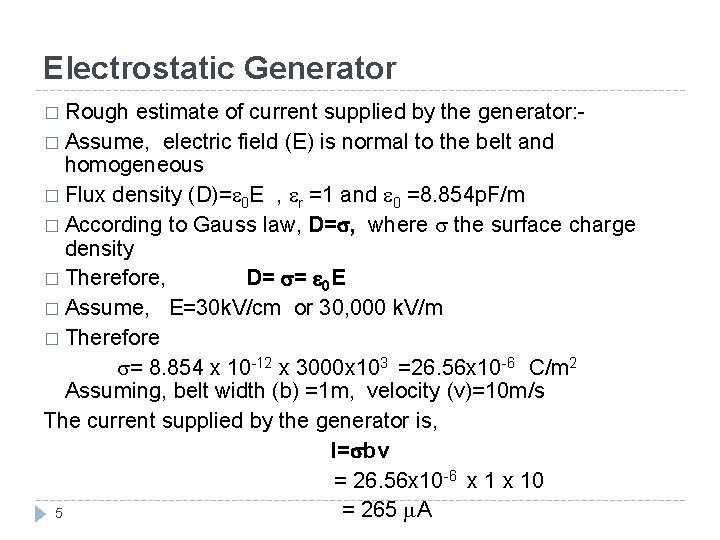Electrostatic Generator � Rough estimate of current supplied by the generator: � Assume, electric field (E) is normal to the belt and homogeneous � Flux density (D)= 0 E , r =1 and 0 =8. 854 p. F/m � According to Gauss law, D= , where the surface charge density � Therefore, D= = 0 E � Assume, E=30 k. V/cm or 30, 000 k. V/m � Therefore = 8. 854 x 10 -12 x 3000 x 103 =26. 56 x 10 -6 C/m 2 Assuming, belt width (b) =1 m, velocity (v)=10 m/s The current supplied by the generator is, I= bv = 26. 56 x 10 -6 x 10 = 265 A 5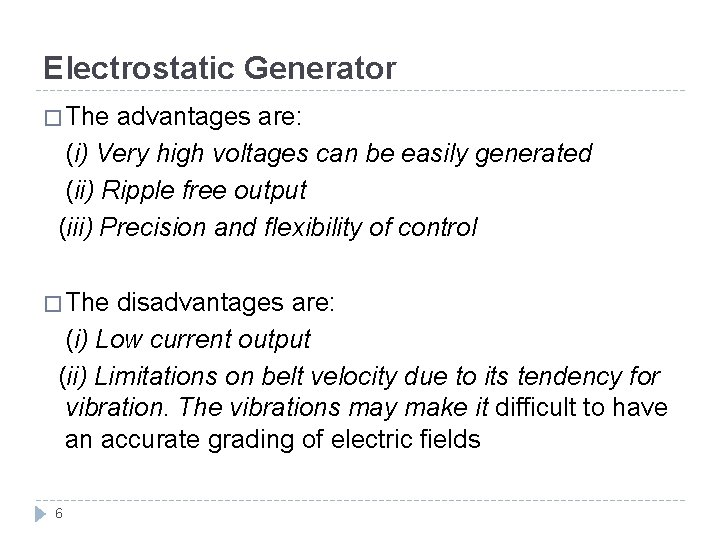Electrostatic Generator � The advantages are: (i) Very high voltages can be easily generated (ii) Ripple free output (iii) Precision and flexibility of control � The disadvantages are: (i) Low current output (ii) Limitations on belt velocity due to its tendency for vibration. The vibrations may make it difficult to have an accurate grading of electric fields 6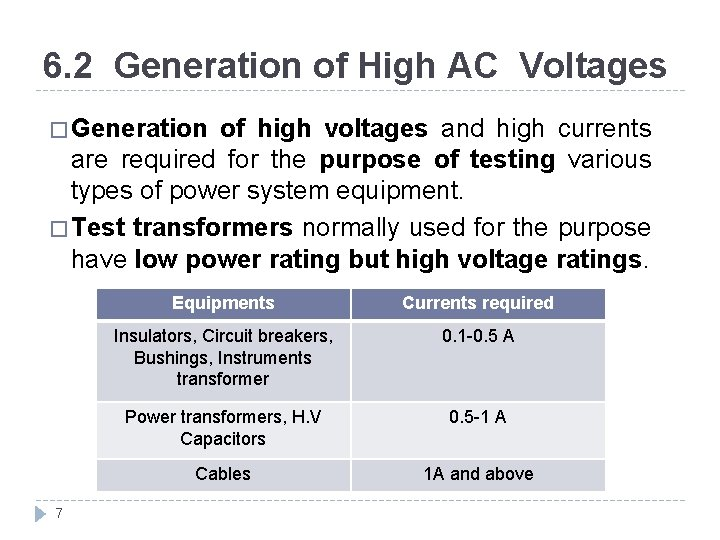6. 2 Generation of High AC Voltages � Generation of high voltages and high currents are required for the purpose of testing various types of power system equipment. � Test transformers normally used for the purpose have low power rating but high voltage ratings. 7 Equipments Currents required Insulators, Circuit breakers, Bushings, Instruments transformer 0. 1 -0. 5 A Power transformers, H. V Capacitors 0. 5 -1 A Cables 1 A and above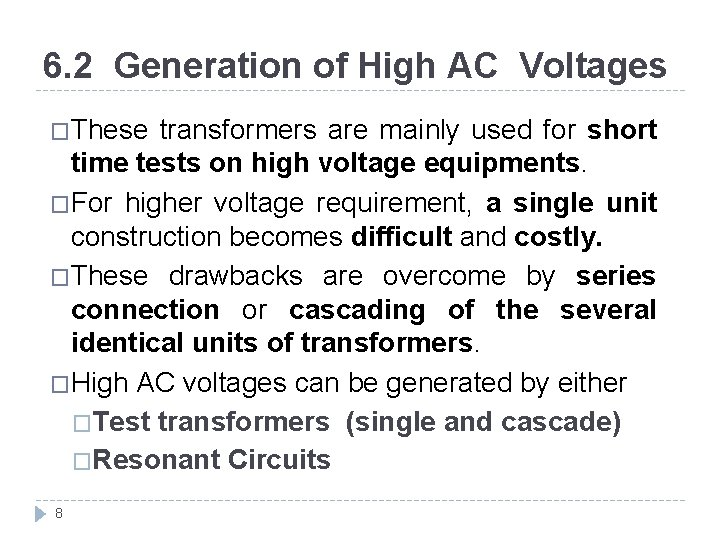6. 2 Generation of High AC Voltages �These transformers are mainly used for short time tests on high voltage equipments. �For higher voltage requirement, a single unit construction becomes difficult and costly. �These drawbacks are overcome by series connection or cascading of the several identical units of transformers. �High AC voltages can be generated by either �Test transformers (single and cascade) �Resonant Circuits 8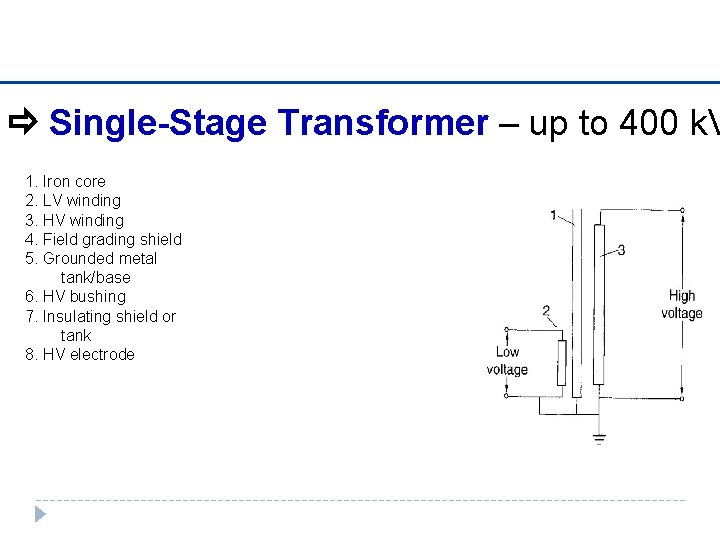Single-Stage Transformer – up to 400 k. V 1. Iron core 2. LV winding 3. HV winding 4. Field grading shield 5. Grounded metal tank/base 6. HV bushing 7. Insulating shield or tank 8. HV electrode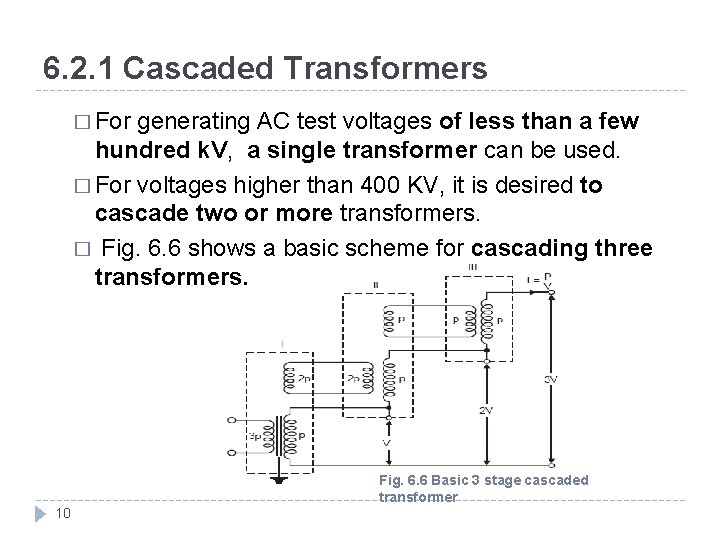6. 2. 1 Cascaded Transformers � For generating AC test voltages of less than a few hundred k. V, a single transformer can be used. � For voltages higher than 400 KV, it is desired to cascade two or more transformers. � Fig. 6. 6 shows a basic scheme for cascading three transformers. Fig. 6. 6 Basic 3 stage cascaded transformer 10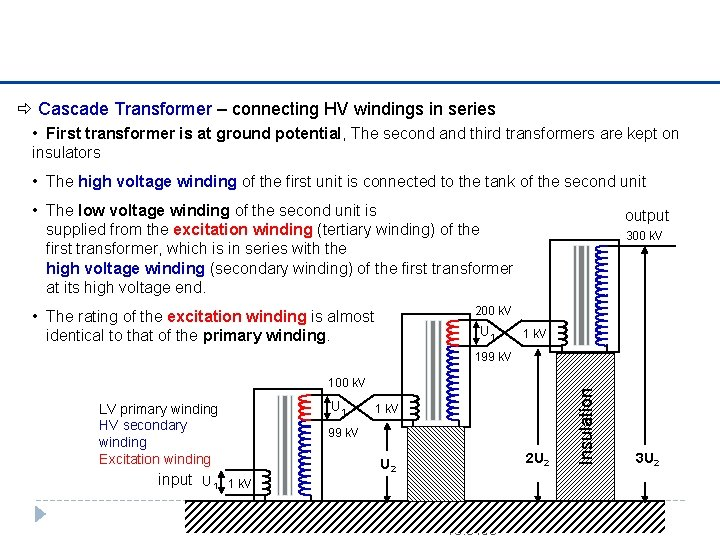Cascade Transformer – connecting HV windings in series • First transformer is at ground potential, The second and third transformers are kept on insulators • The high voltage winding of the first unit is connected to the tank of the second unit • The low voltage winding of the second unit is supplied from the excitation winding (tertiary winding) of the first transformer, which is in series with the high voltage winding (secondary winding) of the first transformer at its high voltage end. output 300 k. V 200 k. V • The rating of the excitation winding is almost identical to that of the primary winding. U 1 1 k. V 100 k. V U 1 LV primary winding HV secondary winding Excitation winding input U 1 1 k. V 99 k. V U 2 2 U 2 Insulation 199 k. V 1 k. V https: //noppa. tkk. fi/noppa/kurssi/s 18. 3150 27/10/2020 3 U 2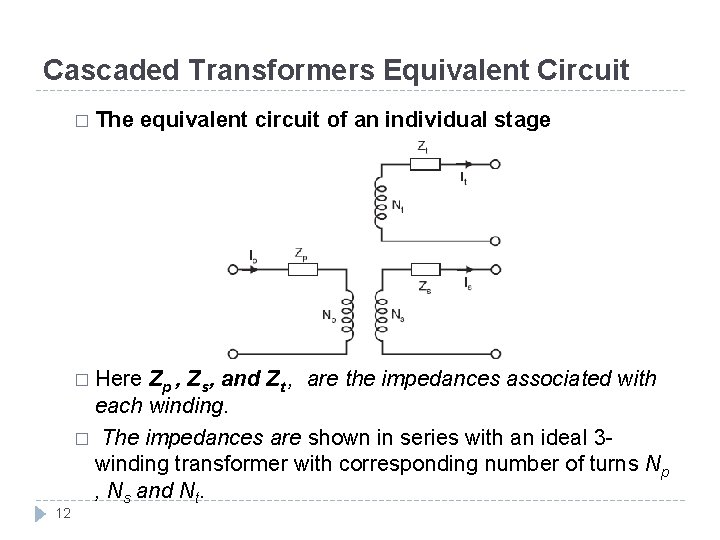Cascaded Transformers Equivalent Circuit � The equivalent circuit of an individual stage � Here 12 Zp , Zs, and Zt , are the impedances associated with each winding. � The impedances are shown in series with an ideal 3 winding transformer with corresponding number of turns Np , Ns and Nt.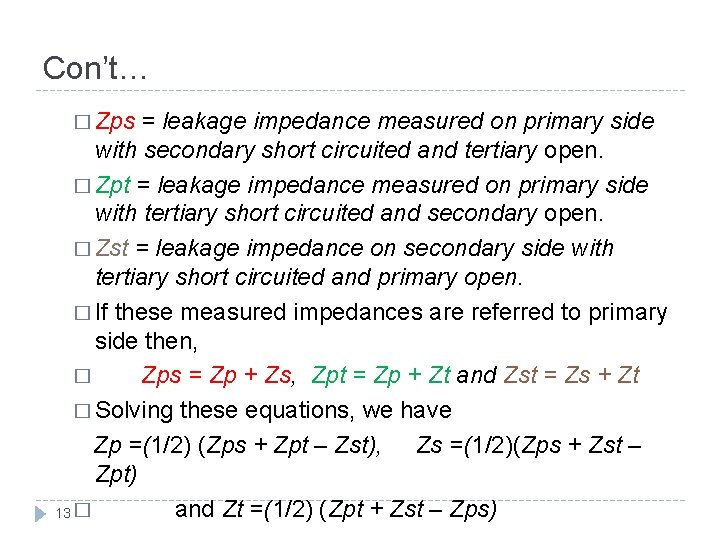Con’t… � Zps = leakage impedance measured on primary side with secondary short circuited and tertiary open. � Zpt = leakage impedance measured on primary side with tertiary short circuited and secondary open. � Zst = leakage impedance on secondary side with tertiary short circuited and primary open. � If these measured impedances are referred to primary side then, � Zps = Zp + Zs, Zpt = Zp + Zt and Zst = Zs + Zt � Solving these equations, we have Zp =(1/2) (Zps + Zpt – Zst), Zs =(1/2)(Zps + Zst – Zpt) and Zt =(1/2) (Zpt + Zst – Zps) 13 �Con’t… � Assuming negligible magnetising current, the sum of the ampere turns of all the windings must be zero. Np Ip – Ns Is – Nt It = 0 � Assuming lossless transformer, we have, Zp = j. Xp, Zs = j. Xs and Zt = j. Xt � Also let Np = Nt for all stages, the equivalent circuit for a 3 -stage transformer would be 14 Equivalent circuit of 3 -stage transformer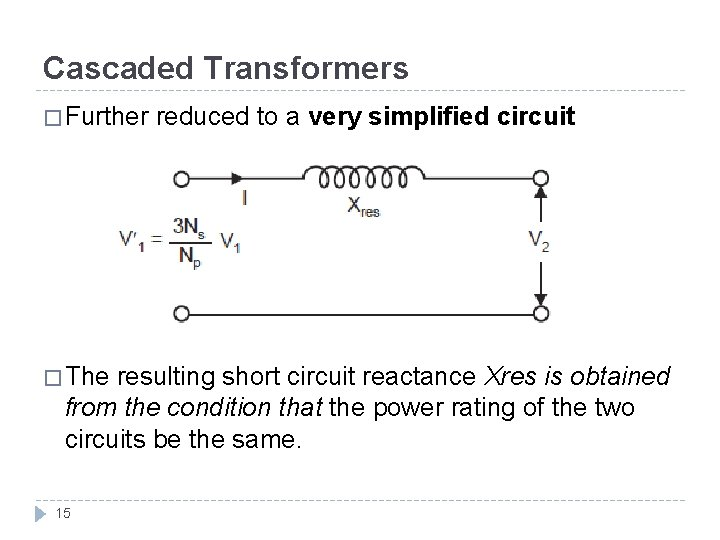Cascaded Transformers � Further � The reduced to a very simplified circuit resulting short circuit reactance Xres is obtained from the condition that the power rating of the two circuits be the same. 15Cascaded Transformers � Here currents have been shown corresponding to high voltage side � Equation above can be generalized for an n-stage transformer as follows: � Where Xpi, Xsi and Xti are the short-circuit reactance of the primary, secondary and tertiary windings of ith transformer. 16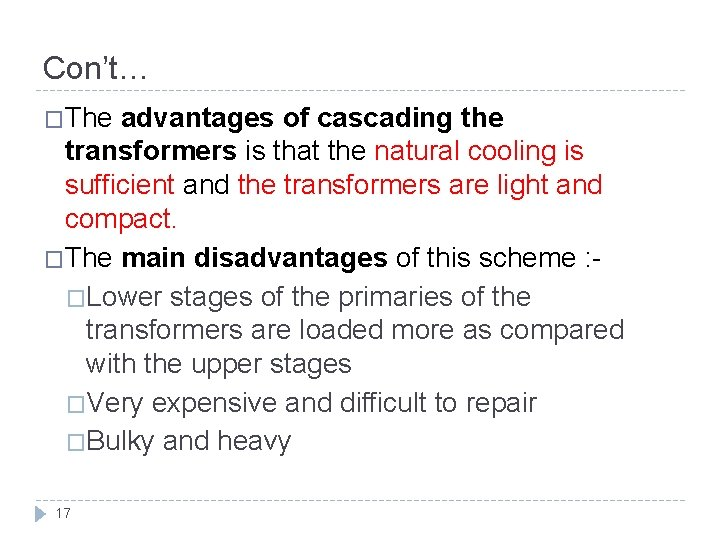Con’t… �The advantages of cascading the transformers is that the natural cooling is sufficient and the transformers are light and compact. �The main disadvantages of this scheme : �Lower stages of the primaries of the transformers are loaded more as compared with the upper stages �Very expensive and difficult to repair �Bulky and heavy 17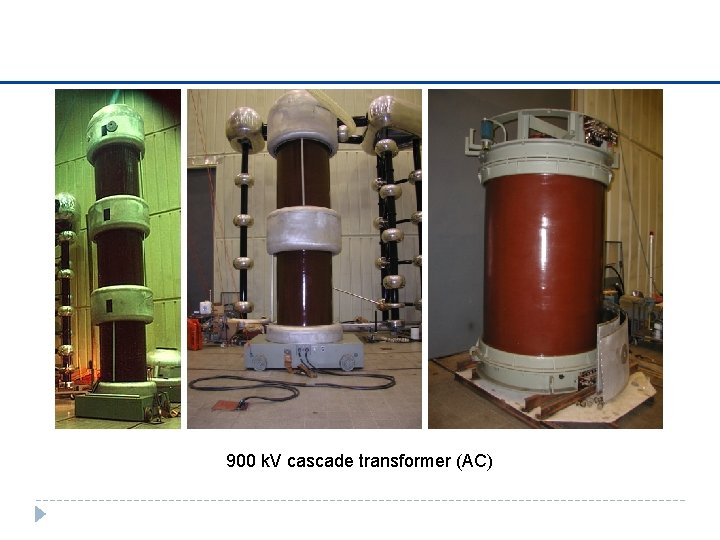900 k. V cascade transformer (AC)6. 2. 2 Resonant Circuit � An alternative method that is more economical and sometimes technically superior is offered by resonant circuits Parallel resonant circuits involves the addition of parallel reactors either in the primary low-voltage circuit or the secondary highvoltage circuit. � The power factor can be greatly improved � A simplified diagram of the parallel resonant test system is given in Fig. 6. 7 � Fig. 6. 7 Parallel resonant test system 19Con’t… � An alternative system is the series resonance circuit. � By resonating the circuit through a series reactor L at the test frequency (50 Hz), harmonics are heavily attenuated. � A simplified diagram of the series resonance test system is given in Fig. 6. 8 Series resonant test system 20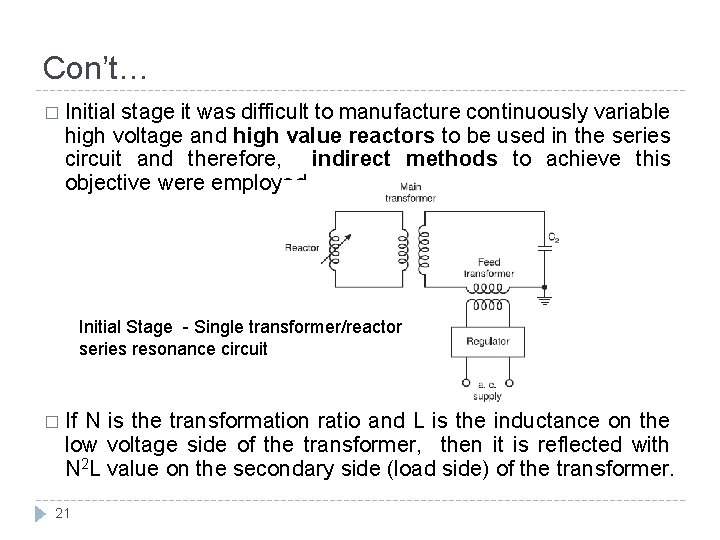Con’t… � Initial stage it was difficult to manufacture continuously variable high voltage and high value reactors to be used in the series circuit and therefore, indirect methods to achieve this objective were employed. Initial Stage - Single transformer/reactor series resonance circuit � If N is the transformation ratio and L is the inductance on the low voltage side of the transformer, then it is reflected with N 2 L value on the secondary side (load side) of the transformer. 21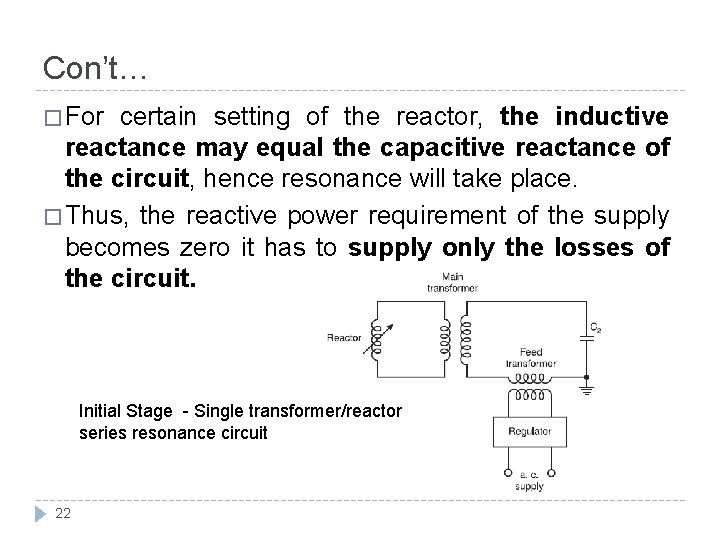Con’t… � For certain setting of the reactor, the inductive reactance may equal the capacitive reactance of the circuit, hence resonance will take place. � Thus, the reactive power requirement of the supply becomes zero it has to supply only the losses of the circuit. Initial Stage - Single transformer/reactor series resonance circuit 22Con’t… � However, the transformer has to carry the full load current on the high voltage side. This is a disadvantage of the method. � The inductor are designed for high quality factors (Q) Q = ωL / R. The feed transformer, therefore, injects the losses of the circuit only. Initial Stage - Single transformer/reactor series resonance circuit 23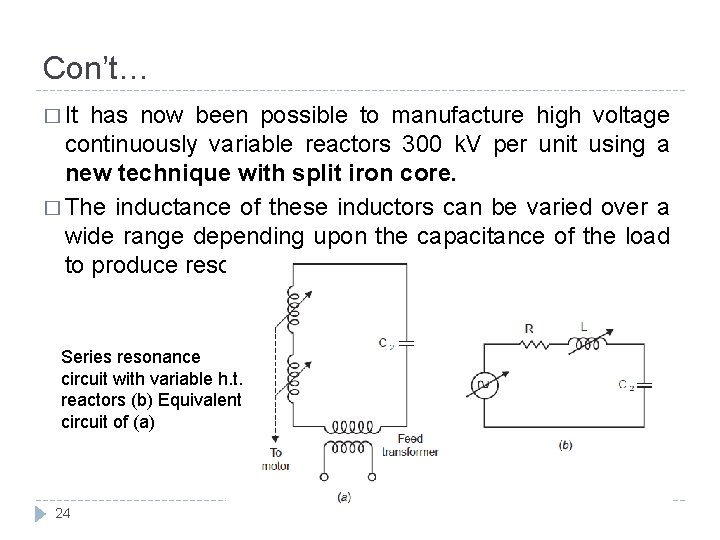Con’t… � It has now been possible to manufacture high voltage continuously variable reactors 300 k. V per unit using a new technique with split iron core. � The inductance of these inductors can be varied over a wide range depending upon the capacitance of the load to produce resonance. Series resonance circuit with variable h. t. reactors (b) Equivalent circuit of (a) 24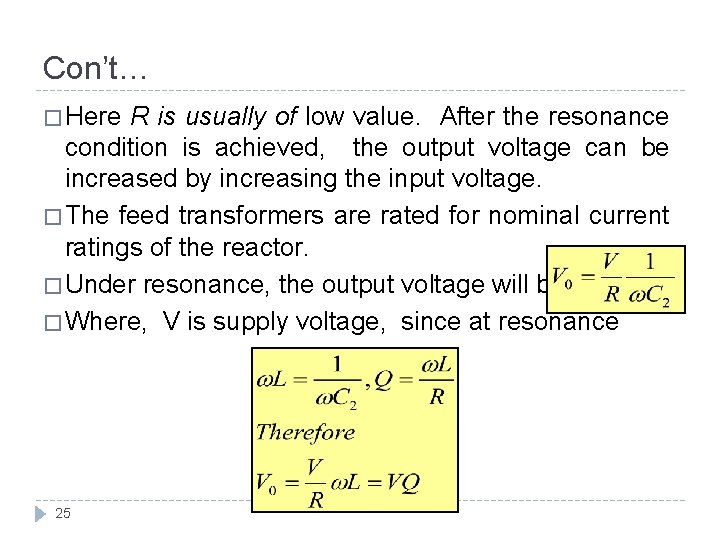Con’t… � Here R is usually of low value. After the resonance condition is achieved, the output voltage can be increased by increasing the input voltage. � The feed transformers are rated for nominal current ratings of the reactor. � Under resonance, the output voltage will be, � Where, V is supply voltage, since at resonance 25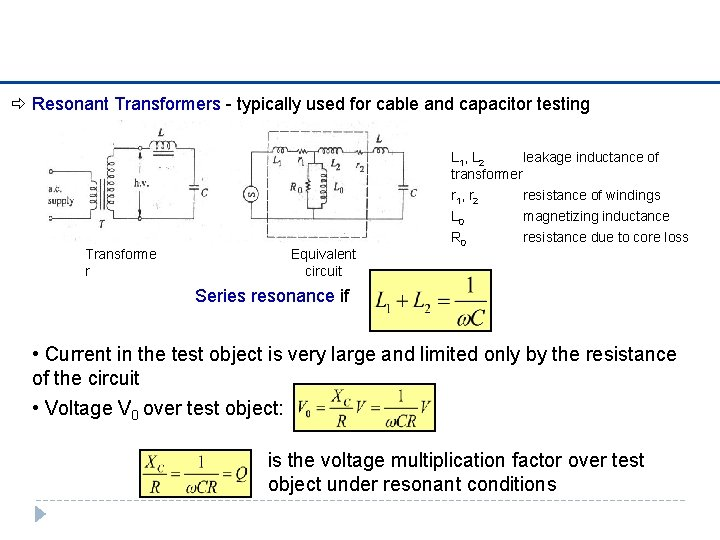Resonant Transformers - typically used for cable and capacitor testing L 1, L 2 leakage inductance of transformer Transforme r Equivalent circuit r 1, r 2 L 0 R 0 resistance of windings magnetizing inductance resistance due to core loss Series resonance if • Current in the test object is very large and limited only by the resistance of the circuit • Voltage V 0 over test object: is the voltage multiplication factor over test object under resonant conditions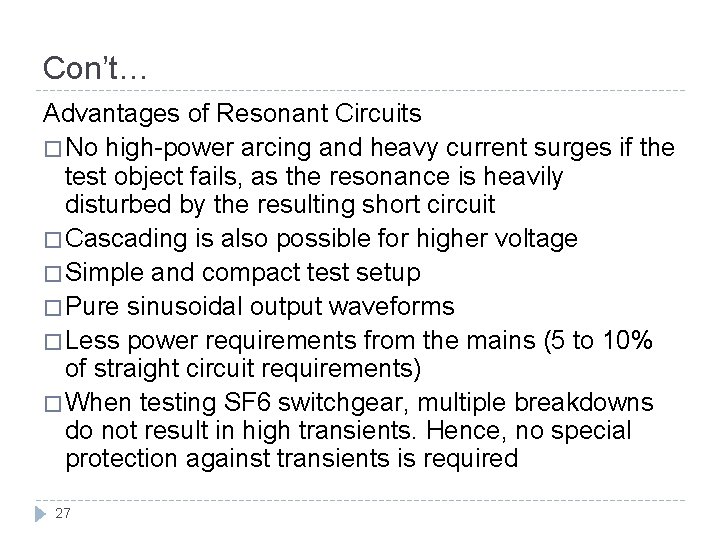Con’t… Advantages of Resonant Circuits � No high-power arcing and heavy current surges if the test object fails, as the resonance is heavily disturbed by the resulting short circuit � Cascading is also possible for higher voltage � Simple and compact test setup � Pure sinusoidal output waveforms � Less power requirements from the mains (5 to 10% of straight circuit requirements) � When testing SF 6 switchgear, multiple breakdowns do not result in high transients. Hence, no special protection against transients is required 27Series resonance transformer